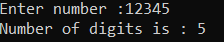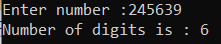# Count Number of Digits in an Integer Number in C#

## Count Number of Digits in an Integer Number in C# with Examples

In this article, I am going to discuss How to Count the Number of Digits in a given integer number in C# with Examples. Please read our previous article, where we discussed How to Count the Number of 1 Bit in a given number in C#.

Example1:
Here, the user will enter an integer value say 432, and our task is to count the numbers of digits present in the number.
Input: 432
Output: 3
Explanation: It has 3 digits 4(1st digit), 3(2nddigit), and 2(3rd digit).

Example2:
Input: 6432
Output: 4
Explanation: It has 4 digits 6(1st digit), 4(2nddigit), 3(3rd digit), and 2(4th digit).

Before moving to the solution part, you should have some basic knowledge of the remainder operator and division operator.

###### Remainder Operator %

The remainder operator % computes the remainder after dividing its left-hand operand by its right-hand operand.

###### Division Operator (/)

The division operator divides the left-hand operand by the right-hand operand and returns the quotient as result. As we are aware of the remainder operator, we know that its returns the remainder of the two operands. If we apply remainder operator with 10 to any number what we will get? The last digit of the number.

Example1:
532 % 10 = 2 || 532 / 10 = 53
53 % 10 = 3 || 53 / 10 = 5
5 % 10 = 5 || 5 / 10 = 0

Example2:
9338 % 10 = 8 || 9338/10 = 933
933 % 10 = 3 || 933/10 = 93
93 % 10 = 3 || 93/10 = 9
9 % 10 = 9 || 9/10 = 0

We are going to apply the same logic to count the number of digits present in the number.

##### Algorithm to Count Number of Digits in an Integer

Step1: Take a variable named number and store the input value entered by the user.
Step2: Take another variable named count to store the count of digits in the number.
Step3: Take a while loop and check for condition (number > 0)
Step4: And in the while block do these two statements
while(number>0)
{
number = number / 10;
count ++;
}

###### Example: C# Program to Count the number of digits in an integer

The following C# Program will count the number of digits in a given integer number.

```using System;
namespace DotNetTutorials
{
public class CountDigitsInNumber
{
static void Main(string[] args)
{
try
{
int number = 0, count = 0;
Console.Write("Enter number :");
while (number > 0)
{
number = number / 10;
count++;
}
Console.WriteLine("Number of digits is : " + count);
}
catch (Exception ex)
{
Console.WriteLine(ex.Message);
}
}
}
}
```
###### Output:##### C# Code (Using function)

In the below C# program, we have created one method to count the number digit in a given integer number.

```using System;
namespace DotNetTutorials
{
public class CountDigitsInNumber
{
static void Main(string[] args)
{
try
{
Console.Write("Enter number :");
int count = FindNumberOfDigit(ref number);
Console.WriteLine("Number of digits is : " + count);
}
catch (Exception ex)
{
Console.WriteLine(ex.Message);
}
}
private static int FindNumberOfDigit(ref int number)
{
int count = 0;
while (number > 0)
{
number /= 10;
count++;
}
return count;
}
}
}
```
###### Output:In the next article, I am going to discuss How to Find the sum of even numbers from 1 to N in C# with Examples. Here, in this article, I try to explain How to Count the Number of Digits in an integer in C# with Examples and I hope you enjoy this How to Count Number of Digits in an integer article.

### 1 thought on “Count Number of Digits in an Integer Number in C# ”

1.using System;

namespace LogicalPrograms
{
public class Program
{
static void Main(string[] args)
{
int Number = 123456734;
string value = Number.ToString();
Console.WriteLine(value.Length);